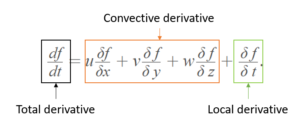# Convective Derivative

The convective derivative, also called a Lagrangian derivative, is commonly used in classical and fluid mechanics and is also found in a wide variety of other sciences such as also astrophysics and cosmology. It is a derivative taken with respect to a coordinate system (e.g., Cartesian, polar, …) moving with velocity u . The derivative is taken with respect to time.

## Convective Derivative vs. Local and Total

The relationship between the local, total, and convective derivatives can be expressed by the equation :The above equation shows that the total derivative is a combination of the convective and local derivatives. The convective derivative, associated with motion, is the first three terms on the right hand side of the above equation. The last term, the local derivative is the change in d with respect to time.

In fluid mechanics the convective derivative is the physical change due to the fluid’s movement from one place to another in the flow field when the flow’s properties are specially different; in other words, the fluid element if on its way to a different place in the flow field where the flow property may be different. The local derivative is the time rate of change at a fixed point. Thus, the total derivative describes the flow property of a changing fluid elements as it moves past a point in the flow .

In steady flows, the local derivative is zero and only the convective derivative makes a contribution .

## References

 Convective Derivative. Retrieved March 4, 2022 from: https://www.chemeurope.com/en/encyclopedia/Convective_derivative.html#:~:text=The%20convective%20derivative%20(also%20commonly,fluid%20mechanics%20and%20classical%20mechanics.
 Jiji, L. (2009). Heat Convection. Springer-Verlag Berlin Heidelberg
 Lui, G. & Liu, M. (2003). Smoothed Particle Hydrodynamics. A Meshfree Particle Method. World Scientific.
 Fluids – Lecture 10 Notes. Retrieved March 4, 2022 from: https://web.mit.edu/16.unified/www/FALL/fluids/Lectures/f10.pdf

CITE THIS AS:
Stephanie Glen. "Convective Derivative" From StatisticsHowTo.com: Elementary Statistics for the rest of us! https://www.statisticshowto.com/convective-derivative/